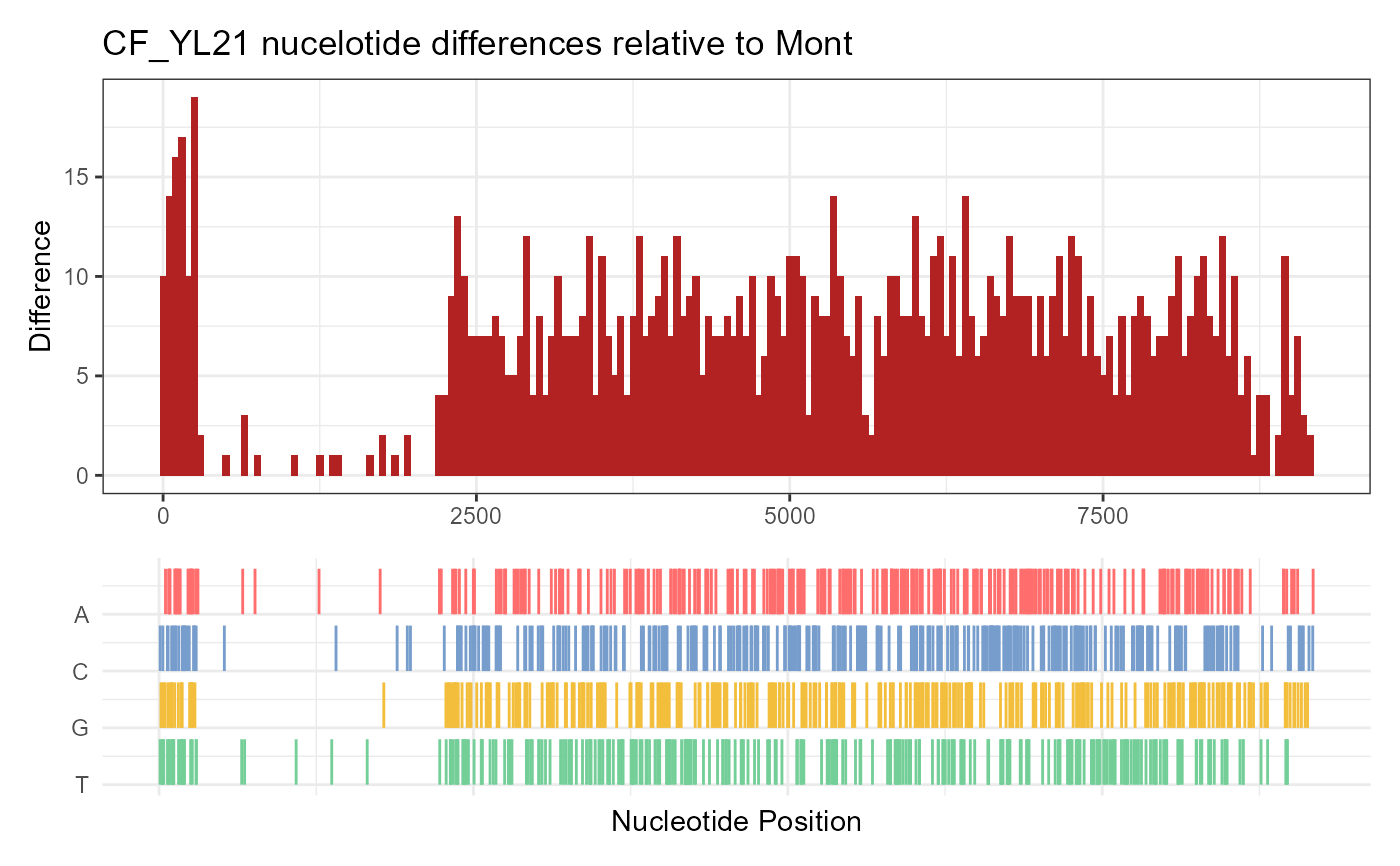plot method for SeqDiff object

# S4 method for SeqDiff,ANY
plot(
x,
width = 50,
title = "auto",
xlab = "Nucleotide Position",
by = "bar",
fill = "firebrick",
colors = c(A = "#ff6d6d", C = "#769dcc", G = "#f2be3c", T = "#74ce98"),
xlim = NULL
)

## Arguments

x SeqDiff object bin width plot title xlab one of 'bar' and 'area' fill color of upper part of the plot color of lower part of the plot limits of x-axis

plot

guangchuang yu

## Examples

fas <- list.files(system.file("extdata", "GVariation", package="ggmsa"),
pattern="fas", full.names=TRUE)
x1 <- seqdiff(fas, reference=1)
plot(x1)